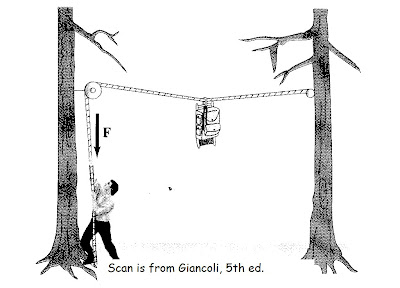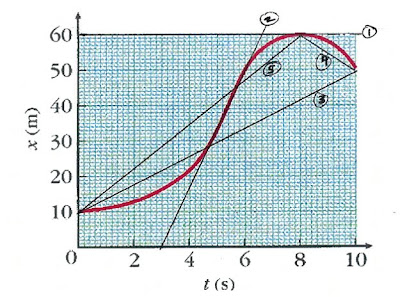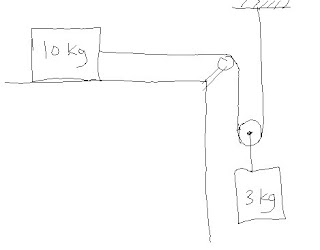Buy that special someone an AP Physics prep book, now with 180 five-minute quizzes aligned with the exam: 5 Steps to a 5 AP Physics 1

Visit Burrito Girl's handmade ceramics shop, The Muddy Rabbit: Yarn bowls, tea sets, dinner ware...

## 17 September 2009

### An equilibrium quizHere's a classic question for the end of the equilibrium unit. I say "classic" because although I got the picture from Giancoli's text, I first encountered the question in Dave Ledden's physics class when I was a senior in high school.

A bear sling, as shown above, is used in some national parks for placing backpackers’ food out of reach of the federal bears. Is it possible to pull the rope hard enough so that it doesn’t sag at all? (Obviously, justify your answer in a couple of sentences… just “yes” or “no” doesn’t cut it :-) )

A good explanation points out that with no sag, no upward force would counteract the bag's weight; thus the bag would not be in equilibrium, and the bag must fall. An even better explanation would explain that the vertical components of the rope's tension provide the upward force to counteract the bag's weight; in order for vertical components of tension to exist, the rope must pull somewhat upward, and so must sag.

An outstanding explanation shows that the vertical components are each Tsinθ, where θ is the angle of the rope measured from the horizontal. If the rope approaches purely horizontal, the angle goes to zero. The vertical equilibrium statement is 2(Tsinθ) = mg. As θ goes to zero, sin θ also goes to zero... meaning that the equilibrium statement for a horizontal rope is mg=0. Impossible!

## 14 September 2009

### Position-time graphs assignment and quizI used the Jerold Touger book for my general physics class for several years. I don't use it anymore -- the writing was way too complicated for my students to read, though I appreciated some of the conceptual treatments and problems. I still use some Touger problems for homework.

Consider the position-time graph in red, representing the motion of a fish. Touger's problem asks a number of questions about average velocity and instantaneous velocity at various times. I assign the problem... and then I give a quiz based on the problem. I drew the five lines in black on the graph, and scanned this graph into the quiz. Here is the quiz, which is based almost verbatim on Touger's posed questions:

A marine biologist shooting video of a rare species of fish uses the video data to produce the graph of the fish’s position x plotted against clock reading t in the graph above.

Velocity is the slope of a position-time graph. For each question, indicate which line on the graph above you should take the slope of in order to answer the question. Mark your choice as:
(A) Line #1
(B) Line #2
(C) Line #3
(D) Line #4
(E) Line #5

1.What is the fish’s average velocity between t = 0 and t = 8 s?

2.What is the fish’s average velocity between t = 8 s and t = 10 s?

3.What is the fish’s average velocity between t = 0 and t = 10 s?

4.What is the fish’s instantaneous velocity at t = 8 s?

5.Estimate the fish’s instantaneous velocity at t = 5 s.

## 11 September 2009

### Mailbag: Forces on a TableFrom Michael Herrin, from Chestatee High School in Gainesville, GA:

I’m having trouble working a problem. It’s from the Cutnell and Johnson’s book chapter 4, and I am curious if you could help point me in the right direction.

Mr. Herrin actually pointed me to a problem in the 5th edition, but I found a slightly different version in the newer edition. In the diagram shown, all pulleys are massless and the surfaces are frictionless. Find the tension in the rope and the acceleration of the masses.

The problem solving process that I teach for Newton’s second law problems is:

1. Draw a free body diagram.
2. Break angled forces into components, if necessary.
3. Write (up-down=ma) and (left-right=ma).This is a two-body problem; therefore, we start with not one but TWO free body diagrams: Look here -->

Note the tricksiness of the diagram for the 3 kg mass. The rope is pulling up TWICE on this mass: once from the left side of the pulley, once from the right side. So we put two tensions on the diagram.

Jacobs’ law of tensions says “One Rope Equals One Tension.” That’s why I didn’t label the tensions T1 and T2, but just T. The tension will be the same throughout.

Now, write Newton’s second law twice, once for each block. The direction of acceleration will be to the right for the 10 kg block, and down for the 3 kg block. (We can see that by imagining releasing the masses from rest, and seeing which way the blocks speed up.)

T = (10 kg)a and (3 kg)g - (T+T) = (3 kg)a

Now, the PHYSICS IS DONE: I have two equations and two unknowns, a and T. Everything else is a known value. All that’s remaining is mathematics.

I think it’s easiest to solve by addition – multiply the first equation by 2, and add the equations together. This cancels out the tension terms; solve to get a = 1.3 m/s2? . Plugging back into either equation, I get T = 13 N. That’s reasonable: the tension is less than the weight of either mass, but is on the same order of magnitude.

Now, ask your students: what would happen to the acceleration if we were to give the 10 kg mass an initial shove to the left. Would the acceleration be greater than, less than, or equal to 1.3 m/s2? Post YOUR answer in the comment section.

GCJ

 Well, okay, sometimes we write (right-left=ma), not (left-right=ma). How to know which is which? Determine the direction of acceleration, and start with that direction.

## 10 September 2009

### The Milk ProblemFinally, yesterday, I got to teach. On the first day, as discussed previously, I get into real physics: equilibrium situations in AP, position-time graphs in general.

I found a new problem appropriate for the first night’s assignment. I want to give students a sense of how physics will be different from math class, but I’m not yet ready to assign conceptual questions about the current topics – we haven’t gotten far enough. I use problems that require serious reasoning, especially those which lend themselves to order-of-magnitude estimates. The following is based on a problem from the Young and Freedman text:

Milk is often sold by the gallon in plastic containers. You are to solve by calculation and reasoning, not through research.

(a) Estimate the number of gallons of milk that are purchased in the United States each year. (Obviously, your answer should include both verbal and mathematical reasoning.)
(b) What approximate weight of plastic does this represent? Compare this weight to something with which you are familiar.

What an excellent question! Even if students WANTED to try to answer through library or google research, that’s a daunting task… as I found out.

My own reasoning: with 300 million people in the USA, figure about a gallon per week for a family of four. That’s around 70 million gallons per week, times 52 weeks, or somewhere near 4 billion gallons of milk per year sold in the US.

A bunch of googling produced
this article from the US General Accounting Office, suggesting that about 7 billion gallons of milk per year were sold in 2001. Hey! I’m well within a factor of 10, which is the goal of such a problem, anyway.

As for the weight of plastic… I originally guessed about 20 g from an empty milk jug, based on my experience with my hanging weights. This gives 108 kg of plastic for the billion gallons sold each year, or about 100,000 tons.

My chemistry colleague, the Atlanta Cracker, Mr. Paul Vickers weighed an empty half-gallon milk carton, getting 47 g. Woodberry Forest Librarian Phoebe Warmack turned up
a claim that nowadays gallon milk jugs are less than 60 g. So my estimate was definitely good enough, because it gives the same ~100,000 tons of plastic as does a weight of 50 g or so.

And this is the whole point of the exercise. Not only do I want my students to gain their first exposure to “Fermi problems” and order-of-magnitude estimation, I also want to make a preemptive strike against the arguments I inevitably hear in class: “You said the answer was 5.9 N, but I got 5.8 N. What did I do wrong?” Or, as always, “isn’t g 9.8, not 10?” Hopefully they will see that a 2% difference is meaningless when making everyday measurements.